Module 14 - A Simple Model of Income Determination Flashcards Preview

Economics > Module 14 - A Simple Model of Income Determination > Flashcards

Flashcards in Module 14 - A Simple Model of Income Determination Deck (48):
1

2

3

4

5

6

7

8

9

Define: Marginal Propensity to Consume (MPC) Average Propensity to Consume (APC) Marginal Propensity to Save (MPS)10

11

12

Explain the Solution to the Simple Model Questions.

Y = C + I where national income (Y) is determined by the sum of consumption expenditures and investment expenditures and C + I = aggregate demand or aggregate expenditure (E). C = bYd where consumption is a function of income. The relationship is proportional at all levels of income, so that APC = MPC = b, I = I0 where the level of investment is fixed exogenously or autonomously, i.e. its value is determined outside the economic system. Where the aggregate demand (expenditure) function lies above the 45deg line aggregate demand is more than the national income, below it is less. At aggregate demand = national income, I=S and the model is in equilibrium.13

Explain the multiplier.

An autonomous increase in aggregate demand (e.g. through increase of investment) has a multiplier effect in the national output and income. Increase in national output = increase in aggregate demand x multiplier Not confined to autonomous change in investment expenditure For the multiplier process to work it’s way to completion, there has to be 1. sufficient unemployment. If the economy were to operate at full employment any autonomous increase in demand would result in inflation as the output Y has reached the potential output. The remaining demand creates and inflationary gap. 2. an MPC between 0 and 1. In the real world calculating the multiplier is very difficult. It therefore is not easy to guide the economy to full employment. - Imported goods have multiplier effect in foreign nations - If increased demand causes demand for money (interest rates) to increase, multiplier may be negative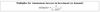14

15

D represents our household’s demand for steak in a week when there are no guests; it shifts to the right D* when guests are coming, the shift being dependent on the number of guests In this example I. the number of guests is an exogenous variable II. price of steak is an exogenous variable III. quantity of steak demanded is an endogenous variable Which of the following is correct? A. I only B. I and III only C. III only D. I, II and III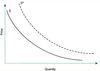16

17

Which of the following is correct?I. When Y = 0, C = a II. When Y = 0, savings = −a III. When Y = Y1, savings = 0A. I only B. II only C. III only D. I, II and III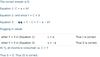18

In the figure I. The MPC is constant II. The APC is constant III. Y = C + S only for income levels > Y1Which of the following is correct? A. I only B. I and II only C. II and III only D. I, II and III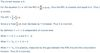1920

An electronics company invested \$10m in new factories, i.e. caused an initial increase in income ΔY of \$10m. Assuming there are sufficient unemployed resources to allow the complete multiplier process to occur, which of the following would the value of the marginal propensity to consume have to be for the total increase in income to be 20 million? A. 0 B. 0.5 C. 1.0 D. 2021

Which of the following numbers (rounded to the nearest whole number) should replace the question marks in Row 4 ΔC and Row 5 ΔY? A. 48 and 32 B. 48 and 40 C. 51 and 48 D. 51 and 41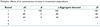22

Which of the following will be the total change in C and Y when the multiplier processes are complete? A. 236 and 336 B. 400 and 500 C. 636 and 836 D. 800 and 100023

If a consumption function is linear and the values of the APC and MPC are equal which of the following is correct? A. The consumption function goes through the origin B. There are unemployed resources in the economy C. The value of the multiplier increases as Y increases D. The value of the multiplier decreases as Y increases24

In economic models the variables whose values are determined I. within the model are known as endogenous variables II. outside the model are known as exogenous variables Which of the following is correct? A. I only B. II only C. Both I and II D. Neither I nor II

he correct answer is C. This is a question about definitions. As a mnemonic try this: outside = ex inside = en Both I and II are true.

2526

Had the consumption function C= bY been indistinguishable from the 45% line which of the following would be true? A. The multiplier would be zero B. Savings and consumption would be equal C. The marginal and average propensity to consume would be equal D. Consumption would not increase as rapidly as income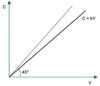27

In the diagram for the consumption function C = bY I. The marginal propensity to consume = b II. The marginal propensity to consume = △C/△Y III. The average propensity to consume = C/Y = b Which of the following is correct? A. I only B. I and III only C. II and III only D. I, II and III28

29

The equation of a consumption function is C= 20 + .8Yd. It can be concluded that I. C will exactly equal Yd when Yd= 100 II. the marginal propensity to consume (MPC) is constant III. the average propensity to consume (APC) is constant Which of the following is correct? A. I only B. I and II only C. II only D. I, II and III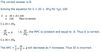30

In a certain community households spend 90¢ out of each additional 1\$ of income. If the local government, wishing to reduce the high local unemployment rate, invested \$500 000 in local schools and parks which of the following is the amount by which total income in the area would increase assuming all multiplier rounds occurred within the area? A. \$500 000 B. \$1 000 000 C. \$1 500 000 D. \$5 000 000.31

If the equation of Aggregate Demand1 is equal to a+ .75Y, which of the following is the autonomous increase in investment required to take the economy from Y1 to YF? A. 750 B. 1000 C. 2000 D. 3000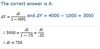32

If the autonomous increase in investment took the aggregate demand curve to Aggregate Demand2, which of the following would happen? A. Some resources would become unemployed B. Inflation would occur C. Potential output would exceed aggregate demand D. The value of the MPC would increase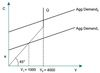The correct answer is B. Had aggregate demand intersected the 45/Q line at the ‘kink’, Y would equal Q, the economy would be operating at capacity/full employment without inflation. For level of demand Aggregate Demand2 all resources will remain employed but aggregate demand will exceed Q and inflation will occur. Thus A and C are wrong and B is correct. Assuming Aggregate Demand2 is parallel to Aggregate Demand1, the slope of the consumption function and consequently the value of the MPC will be unaltered. Thus D is incorrect.

33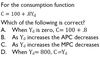34

Which of the following is correct? The marginal propensity to consume out of disposable income A. is sometimes positive and sometimes negative. B. varies between 0.20 and 1.25. C. cannot be calculated from the data provided. D. is 0.8.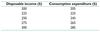The correct answer is D. The marginal propensity to consume (MPC) is calculated by dividing the change in consumption by the accompanying change in disposable income, i.e. ΔC/ΔY. As disposable income increases, the accompanying changes in consumption and the marginal propensity to consume are as follows: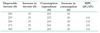35

Which of the following is correct? The average propensity to consume A. is constant. B. increases as disposable income increases. C. decreases as disposable income increases. D. has a value less than 1.The correct answer is C. The average propensity to consume of disposable income is found by dividing consumption expenditure by disposable income. At the lowest level, the value of APC exceeds 1 but declines to a value of 0.95 as income increases.36

Which of the following is correct? A straight-line consumption function with a positive intercept (cuts the vertical axis above zero) and a slope of less than 1 (slopes upward to the right at less than 45° angle) would be one for which A. the average propensity to consume increases with the level of income. B. the average propensity to consume is constant for all levels of income. C. the marginal propensity to save is positive at all levels of income. D. the average propensity to save is positive at all levels of income.

The correct answer is C. Figure A2.15 represents the consumption function described and the savings function derived from the consumption function. Consumption increases at a lower rate than income; as a result the average propensi-ty to consume, C/Y, falls as income rises. Thus, responses A and B are incorrect. For income levels less than y1, consumption exceeds income, so that dissaving takes place. Thus the average propensity to save is negative for income levels less than y1, is equal to zero at y1, and is positive thereafter. Thus response D is incorrect. The marginal propensity to save, S/Y, is given by the slope of the savings function and equals 1 − MPC, since MPC + MPS = 1. Like MPC, MPS is constant and positive for all levels of income.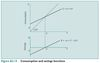37

For a certain economy, the consumption function is C = 15 + 0.75 Yd (where Yd = disposable income). In addition, I. saving = 10 when disposable income = 100. II. consumption = 240 when disposable income = 300. III. a level of disposable income exists for which consumption = saving. Which of the following is correct? A. I only. B. I and II only. C. II and III only. D. III only.

The correct answer is B. If C = 15 + 0.75Yd, then when Yd = 100 C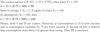38

39

40

41

42

43

C = 50 + 0.5Y I = 100 Y ≡ C + I What is the equilibrium value of Y? A. 50. B. 100. C. 250. D. 300.4445

What would be the equilibrium value of C if I were to increase from 100 to 200? A. 200. B. 300. C. 400. D. 500.46

What is the change in the value of the investment multiplier when I changes from 100 to 200? A. It remains constant at 2. B. It increases from 2 to 3. C. It doubles from 2 to 4. D. It remains constant at 0.5.47

If I became zero, what would the equilibrium value of C be? A. 0. B. 0.5Y. C. 50. D. 100.48

The consumption function in Figure 14.8 relates household consumption expenditure (C) to disposable income (Yd). It shows I. a constant marginal propensity to consume (MPC). II. a constant average propensity to consume (APC). III. dissavings for Yd < \$4000. Which of the following is correct? A. I only. B. I and III only. C. II only. D. I, II and III.